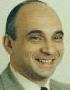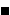# Cut The Knot!

An interactive column using Java applets
by Alex Bogomolny

December 2002I have heard Mr Hobbes say, that he was want to draw lines on his thigh and on the Sheets, abed, and also multiply and divide. He would often complain that Algebra (though of great use) was too much admired, and so followed after, that it made men not contemplate and consider so much the nature and power of Lines, which was a great hindrance to the Groweth of Geometrie... John Aubrey Brief Lives, Penguin Books, 2000, p. 428 (also, Fauvel, p. 307)

The mathematical object that goes by the name of complete quadrilateral is neither complete nor quadrilateral, at least not in the sense in which the word "quadrilateral" appears in, say, Brahmagupta's theorem about cyclic quadrilaterals. Simply put, it's not a 4-sided polygon. (The latter is sometimes called a simple quadrilateral.) Seldom mentioned in elementary courses, it plays an important role in projective geometry, where it is used for a ruler only construction of harmonic conjugates and as a corner stone of the projective coordinate system [Möbius, p. 91, Kline, p. 127-128, Courant, p. 179].

A complete quadrilateral is a configuration of four straight lines in general position and six points at which the lines intersect. This is the (projective) configuration that Hilbert and Cohn-Vossen used to denote as (6243) [Hilbert, p. 96] meaning a system of 6 points and 4 lines, 2 lines through each point, 3 points per line. A triangle in this notations appears as (3232) and has the property that the straight lines joining the points of the configuration are necessarily the lines of the configuration. This is obviously not the case with the configuration (6243). There are three lines joining points of the configuration that do not count among the four configuration lines. (These are known as the diagonals of the complete quadrilateral.) This is why the configuration may be called incomplete. Adjoining the three lines to the configuration does not save the situation because such an operation adds new points of intersection. Adding them leaves room for new lines and so on. In fact, all but trivial configurations are incomplete.

A typical quadrilateral (4-sided polygon) is represented by the symbol (4242) and is therefore a typical quadrangle (4-angled polygon). However, the complete quadrangle is the configuration dual to (6243), i.e., (4362), which is the configuration of 4 points and 6 straight lines, 2 points on a line, 3 lines through a point.

In plane geometry, there are quite a few curious theorems associated with the complete quadrilateral [Wells, p. 34-35], some of which are illustrated by the applet below.

1. First of all, we have the Theorem of Complete Quadrilateral: the midpoints of the three diagonals are collinear.

2. Next we consider the four triangles formed by the four lines (omitting one of them at a time.) The orthocenters of the triangles are collinear and the line (Ortholine in the applet) is perpendicular to the line (Midline in the applet) of the three mid-diagonals. (This is known as the Gauss-Bodenmiller theorem.)

3. Also, the ortholine serves as the common radical axis of the three circles constructed on the diagonals as diameters, such that whenever the circles intersect, all three of them intersect in two points on the ortholine.

4. The circumcircles of the four triangles meet in a point, the Miquel point of the complete quadrilateral.

The practice of omitting one of the given lines is reminiscent of Clifford's chain and Frank Morley's research. The complete quadrilateral is nothing but a 4-line in Morley's terminology. In a 1903 paper he showed that

1. the perpendiculars from the 9-point centers of the four triangles to the respective lines omitted from the 4-line in order to obtain the triangles, meet in a point. The common point lies on the ortholine.

(In the applet, the four lines are each defined by two draggable points. Dragging one of the points rotates the line around the other. The line may be also translated by dragging it anywhere away from the points. The four triangles are also shown in translated positions to avoid cluttering the diagram.)

13 July 2015, Created with GeoGebra

Finally, the applet helps verify a problem that appeared in the latest issue of Monthly (Dec 2002, v 109, N 10, p. 921):

10980. Proposed by Ye Zhong Hao, Shanghai Educational Press, Shanghai City, China and Wu Wei Chao, Guang Zhou University(New), Guang Zhou City, China. Consider four distinct straight lines in the same plane, with the property that no two of them are parallel, no three are concurrent, and no three form an equilateral triangle. Prove that, if one of the lines is parallel to the Euler line of the triangle formed by the other three, then each of the four given lines is parallel to the Euler line of the triangle formed by the other three. (The Euler line passes through the centroid, circumcenter and orthocenter of a triangle.)

Now, the condition that none of the triangles involved is equilateral seems artificial (except, of course, for the uncertainty of the presence of the Euler line in equilateral triangle.) Indeed, in the remaining portion of the column, I shall outline a proof of a simplified statement:

1. If the Euler line of one of the four triangles is parallel to the respective omitted line, the same holds true of the remaining three triangles.

Which is a nice addition to the "Complete Quadrilateral" collection. First observe the effect of translation of one of the lines on the four triangles. When one of the lines is translated, one of the triangles remains fixed, while the other three undergo a homothetic transformation. But a homothety maps a line (Euler's in particular) onto a parallel line.

Thus to start with, translate one of the lines of the configuration until it passes through one of the configuration points it was not incident with before. The configuration (see the applet below) is no longer the complete quadrilateral, but consists of two "Siamese" triangles that share the baseline and one of the sides. (Check the "Show names" box.) We can see in fact three such pairs of twins --(ABC, ACD) being one pair -- or a set of triplets: ABC, ACD, ABD.

The original problem is equivalent to showing that if the Euler line of ΔABC is parallel to AD, then the Euler line of ΔACD is parallel to AB.

### This applet requires Sun's Java VM 2 which your browser may perceive as a popup. Which it is not. If you want to see the applet work, visit Sun's website at https://www.java.com/en/download/index.jsp, download and install Java VM and enjoy the applet.

A key observation here is that wherever the Euler lines appear to be parallel to the corresponding triangle side lines, they cross on the common baseline. To ascertain whether this is indeed the case, note that on the Euler line there is a slew of remarkable points [Kimberling, p. 128]. Of course any two determine the line uniquely. As in the problem statement, I shall consider the three most important: the centroid, the circumcenter and the orthocenter of a triangle.

The next applet illustrates the following

### Proposition

Given ΔABD and a point C on BD. Through the centroid (circumcenter, orthocenter) of ΔACD draw a line parallel to AB. Similarly, draw a line parallel to AD through the centroid (circumcenter, orthocenter) of ΔABC. Then the two lines meet on BD.13 July 2015, Created with GeoGebra

Assuming the proposition true, it follows that if a line parallel to AB is drawn through, the centroid (circumcenter, orthocenter) of ΔACD to its intersection with BD and the latter point is joined to the centroid (circumcenter, orthocenter) of ΔABC, then the resulting line is parallel to AD.

Therefore, if the line parallel to AB happens to be the Euler line of ΔACD, then the three lines drawn from its intersection with BD to the centroid, circumcenter, and orthocenter of ΔABC are all parallel to AD and hence coalesce into the Euler line of that triangle. This proves our simplified version of the problem.

### Proof of Proposition

I'll use the dynamic approach that worked for us so well with another Monthly problem. Let's fix ΔABD, but allow point C glide over BD. With each position of C, we associate two lines: one parallel to AB, the other to AD passing through the denominationally corresponding points of triangles ACD and ABC. For the centroid, the circumcenter and the orthocenter it is quite clear that if the lines intersect on BD for one position of C, then the same is true for all other positions as well. (What is lost or gained on the left from the common point of intersection, is gained or lost on its right.)

Thus the problem is reduced to finding a position for C, for which the claim is obvious. For the centroid we may take C to be the midpoint of BD. The lines in question then will be the midlines of ΔABD drawn from C. For the circumcenter and the orthocenter C could be taken to be the foot of the altitude from A. Then both ΔABC and ΔACD are right. Their circumcenters lie on AB and AD, respectively, such that the lines in question are again the midlines of ΔABD. As regard the lines through the orthocenter, the situation is even simpler, since in this case, the orthocenters of both triangles ABC and ACD lie at C.Property #6 has a very nice

### Corollary

Assume the Euler line of ΔABC intersects side AB in M and side AC in N. Then the Euler line of ΔANM is parallel to BC.

### References

1. J. Aubrey, Brief Lives, Penguin Books, 2000
2. R. Courant and H. Robbins, What is Mathematics?, Oxford University Press, 1996
3. J. Fauvel, J. Gray (eds), The History of Mathematics. A Reader, The Open University, 1987
4. J. Fauvel et al (eds), Möbius and His Band, Oxford University Press, 1993
5. D. Hilbert and S. Cohn-Vossen, Geometry and Imagination, Chelsea Publishing Co, NY 1990.
6. C. Kimberling, Triangle Centers and Central Triangles, Utilitas Mathematica Publishing Inc., 1998
7. M. Kline, Mathematical Thought from Ancient to Modern Times, Oxford University Press, 1972
8. F. Morley, Orthocentric Properties of the Plane n-Line, Trans Amer Math Soc, 4 (1903) 1-12.
9. D. Wells, Curious and Interesting Geometry, Penguin Books, 1991•